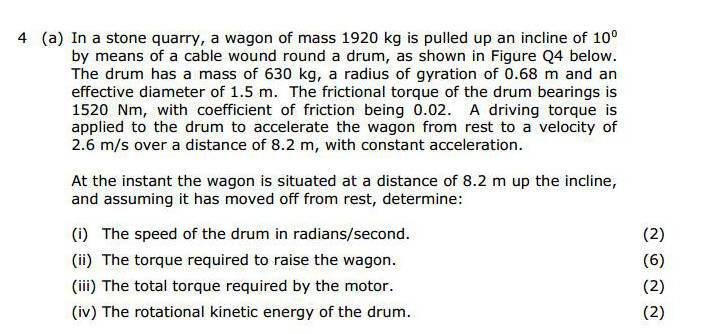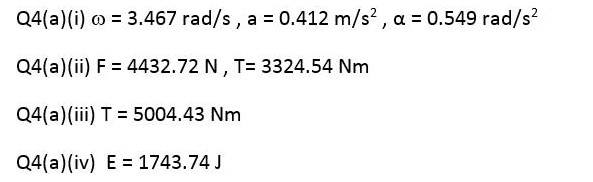# Force/Slope QuestionI've worked out (i), having problems with (ii). I know that the torque will be force x 0.75 but I haven't had any success in working out the force in the first place.

Bystander
Homework Helper
Gold Member
What's the force required to accelerate the wagon if there's no incline?

What's the force required to accelerate the wagon if there's no incline?

Without an acceleration, I'm not sure.

Bystander
Homework Helper
Gold Member
Without an acceleration, I'm not sure.
From the problem statement, "... accelerate the wagon from rest to 2.6 m/s over a distance of 8.2 m at constant acceleration ...."

From the problem statement, "... accelerate the wagon from rest to 2.6 m/s over a distance of 8.2 m at constant acceleration ...."

Right I've got the acceleration but I'm not getting the correct force. You're saying the force needed to work out the torque is when there is no incline?

Bystander
Homework Helper
Gold Member
when there is no incline?
No. You've got the force to do the acceleration? Can you calculate the force necessary to move the wagon up the incline? Add them.

No. You've got the force to do the acceleration? Can you calculate the force necessary to move the wagon up the incline? Add them.

F = ma
= 1920 x 0.41
= 787.2

Fw = mgsinangle
= 1920 x 9.81 x sin10
= -10246.7

Bystander
Homework Helper
Gold Member
= 1920 x 9.81 x sin10
= -10246.7
Don't just punch numbers into a calculator and blindly accept the number that comes out. What's the sin of 10°?

Don't just punch numbers into a calculator and blindly accept the number that comes out. What's the sin of 10°?

Fw = mgsinangle
= 3270.7

Bystander
Homework Helper
Gold Member
Better.

•smr101
Better.

Then just add the Ff to that and the Fu I've got it... Thanks, I've made that much more difficult than it should have been!

I'm assuming the torque needed for the drum, which would be used to calculate the total torque needed by the motor is the same? Just different values.